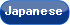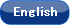Please use this identifier to cite or link to this item: `http://hdl.handle.net/10119/10903`

 Title: Chapter VII: Gödel-Dummett Logic Authors: Baaz, MatthiasPreining, Norbert Keywords: fuyyz logicGödel logicintermediate logic Issue Date: 2011-12-21 Publisher: College Publications Magazine name: Handbook of Mathematical Fuzzy Logic Volume: 2 Start page: 585 End page: 626 Abstract: The logics we present in this chapter, Gödel logics, can be characterized in a rough-and-ready way as follows: The language is a standard (propositional, quantified propositional, first-order) language. The logics are many-valued, and the sets of truth values considered are (closed) subsets of [0, 1] which contain both 0 and 1. 1 is the ‘designated value,’ i.e., a formula is valid if it receives the value 1 in every interpretation. The truth functions of conjunction and disjunction are minimum and maximum, respectively, and in the first-order case quantifiers are defined by infimum and supremum over subsets of the set of truth values. The characteristic operator of Gödel logics, the Gödel conditional, is defined by a → b = 1 if a <= b and = b if a > b. Because the truth values are ordered (indeed, in many cases, densely ordered), the semantics of Gödel logics is suitable for formalizing comparisons. It is related in this respect to a more widely known many-valued logic, Łukasiewicz (or ‘fuzzy’) logic (see Chapter V– although the truth function of the Łukasiewicz conditional is defined not just using comparison, but also addition. In contrast to Łukasiewicz logic, which might be considered a logic of absolute or metric comparison, Gödel logics are logics of relative comparison. There are other reasons why the study of Gödel logics is important. As noted, Gödel logics are related to other many-valued logics of recognized importance. Indeed, Gödel logic is one of the three basic t-norm based logics which have received increasing attention in the last 15 or so years (the others are Łukasiewicz and product logic; see). Yet Gödel logic is also closely related to intuitionistic logic: it is the logic of linearly-ordered Heyting algebras. In the propositional case, infinite-valued Gödel logic can be axiomatized by the intuitionistic propositional calculus extended by the axiom schema (A → B) ∨ (B → A). This connection extends also to Kripke semantics for intuitionistic logic: Gödel logics can also be characterized as logics of (classes of) linearly ordered and countable intuitionistic Kripke structures with constant domains . Furthermore, the infinitely valued propositional Gödel logic can be embedded into the box fragment of LTL in the same way as intuitionistic propositional logic can be embedded into S4. Rights: Copyright (C) 2011 College Publications. Matthias Baaz, Norbert Preining, "Chapter VII: Gödel-Dummett Logic", Handbook of Mathematical Fuzzy Logic, Volume 2, 2011, pp.585-626. This material is posted here with permission of the College Publications. URI: http://hdl.handle.net/10119/10903 Material Type: publisher Appears in Collections: z4-10-2. 図書 (Book, Book Chapter)

Files in This Item:

File Description SizeFormat